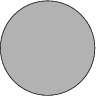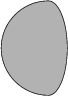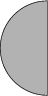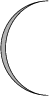Mathematical and Physical Journal
for High Schools
Issued by the MATFUND Foundation
 Already signed up? New to KöMaL?# Exercises and problems in InformaticsSeptember 2003

I. 55. An integer n>1 is said to be highly composite, if the number of its divisors is greater than those of any positive integers smaller than n.

Write your program (i55.pas, ...) that displays all highly composite numbers up to a given positive integer N (1$\displaystyle \le$N $\displaystyle \le$1 000 000).
(10 points)

I. 56. Write a program (i56.pas, ...) which draws a given phase of the Moon. Full Moon is represented as a filled circle, while New Moon should be displayed as an empty circle centered at the middle of the screen and with radius of 100 pixels. The parameter of your program should be the diameter of the crescent defined according to the Figures.
(10 points)200 140 100 10

I. 57. A polynomial P(x) of degree n is given by its coefficients P(x)=a0+a1 x+a2 x2+...+an xn. The derivative of P(x) is the following polynomial of degree n-1

P'(x)=a1+2a2 x+3a3 x2+...+n an xn-1.

Further derivatives of this polynomial can be computed until all of its coefficients become zero.

Prepare your sheet (i57.xls) that produces the derivatives of a polynomial of degree at most 10 as shown in the Figure.
(10 points)

 P(x)= 1 + 1 x1+ 1 x2+ 1 x3+ 1 x4+ 1 x5+ 1 x6+ 1 x7+ 1 x8 1 1 + 2 x1+ 3 x2+ 4 x3+ 5 x4+ 6 x5+ 7 x6+ 8 x7+ 0 x8 2 2 + 6 x1+ 12 x2+ 20 x3+ 30 x4+ 42 x5+ 56 x6+ 0 x7+ 0 x8 3 6 + 24 x1+ 60 x2+ 120 x3+ 210 x4+ 336 x5+ 0 x6+ 0 x7+ 0 x8 4 24 + 120 x1+ 360 x2+ 840 x3+ 1680 x4+ 0 x5+ 0 x6+ 0 x7+ 0 x8 5 120 + 720 x1+ 2520 x2+ 6720 x3+ 0 x4+ 0 x5+ 0 x6+ 0 x7+ 0 x8 6 720 + 5040 x1+ 20160 x2+ 0 x3+ 0 x4+ 0 x5+ 0 x6+ 0 x7+ 0 x8 7 5040 + 40320 x1+ 0 x2+ 0 x3+ 0 x4+ 0 x5+ 0 x6+ 0 x7+ 0 x8 8 40320 + 0 x1+ 0 x2+ 0 x3+ 0 x4+ 0 x5+ 0 x6+ 0 x7+ 0 x8